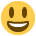In Ordering API there are requests that bring large amounts of data such as businesses, products, orders or users that can be an array of thousands or millions of objects that make the request heavy and consequently slow. For this our team has added that the data can be filtered with one simple parameter:

• where: This parameter is a JSON it serves to filter request by specifics attributes, and this will only return the elements that have the attributes exactly the same as the scripts in the "where". For example, if the user request is made, and the `where = {" level ": 4}` is added, the response will only have level 4 users.
``````{
"city_id": 1,  //filter for users with city_id equals to 1
"enaled": true, //also can be added anothers attriibuttes to filter this works as AND
"level": [2,3] //Elements also can be filter by diferents values for the same attribute and these should be in an array
}
``````

To have a better idea of how it works, we will give an example where we only want to obtain the orders 2, 3 and 5 then we make the request in the following way:

• `http://127.0.0.1:8080/v400/en/development/orders?mode=dashboard&where={"id":[2,3,5]}&params=id,business_id,customer_id`

Here we request the orders id with an array (where={"id":[2,3,5]})
And we will get the following response.

``````{
"error": false,
"result": [
{
"id": 2,
"customer_id": 2
},
{
"id": 3,
"customer_id": 2
},
{
"id": 5,
"customer_id": 2
}
]
}
``````

From now on on the documentation you will find which data can be whereable with the attribute whereable.

## The WHERE V2

In order not to be limited to the fact that the value is only equal, the ordering team has implemented more forms of comparison. now instead of an object, the where is an array and the value attribute can be an object to assign the conditions and if not it will be the equal by default.
the condicion can be de usual comparative (>, <, <=, >=, =) or the comparative "ilike" to look for something similar.

### 📘

every single Objects work as AND, OR is not implemented yet.

``````[
{
"attribute": 'attribute',
"value": {
"condition": '>',
"value": 'value'
}
},
{
"attribute": 'attribute',
"value": {
"condition": 'ilike',
"value": 'value'
}
},
{
"attribute": 'attribute',
"value": 'value'
}
]
``````

## The new WHERE

Now Ordering team have implemented a "Where" where the OR can be used with a friendly structure and a nested conditions can be build``````{
"conditions": [
{
"attributte": "attribute",
"value": "value"
},
{
"conector": "AND",
"conditions": [
{1},
{2}
]
}
],
"conector": "OR"
}
// WHERE (attribute = "attribute") OR ({1} AND {2}), 1 and 2 are only for short example should have de attribute value structure
``````

## Using Ordering SDK

For this behavior there is the `where()` method, which is sent an array conditions that you want to bring.
Example:

``````const where = [
{
"attribute": 'id',
"value": {
"condition": '=',
"value": [2,3,5]
}
},
]
const response = await ordering.orders().where(where).get()
``````